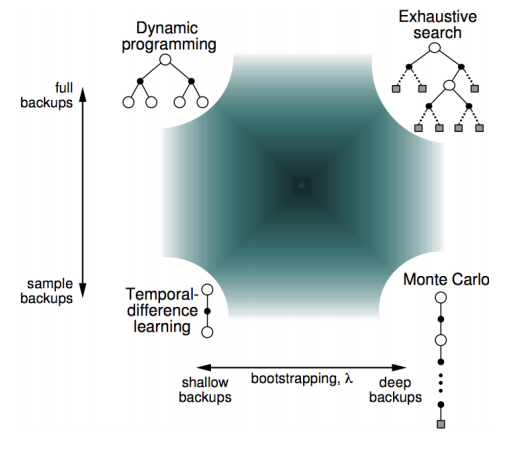# Temporal Difference Learning

## n-Step Return

Consider the following n-step returns for $n=1, 2, \infty$:

• 1-step return ($\approx \text{TD(0)}$): $G_t^{(1)} = r_t + \gamma V^{\pi}(s_{t+1})$
• 2-step return: $G_t^{(2)} = r_t + \gamma r_{t+1} + \gamma^2 V^{\pi}(S_{t+2})$
• n-step return: $G_t^{(n)} = r_t + \gamma r_{t+1} + \cdots + \gamma^{n-1} r_{t+n-1} + \gamma^{n}V^{\pi}(s_{t+n})$
• $\infty$-step return ($= \text{MC}$): $G_t^{(\infty)} = r_t + \gamma r_{t+1} + \cdots + \gamma^{T} r_T (\approx G_{i, t})$

$\rarr$ n-step temporal difference learning $V^{\pi}(s_t) = V^{\pi}(s_t) + \alpha ( \underbrace{G_t^{(n)}}_{\text{TD target}} - V^{\pi}(s_t))$

## Combines Information from many different time-steps

In Temporal difference learning, estimated returns are collected. Then, we can efficiently combine information from all time-steps. The $\lambda$-return ($G_t^{\lambda}$) combines all n-step returns $G_t^{(n)}$ with weight $(1-\lambda) \lambda^{n-1}$:
(Assume that termination step is $T$)

\begin{aligned} G_t^{\lambda} &= (1- \lambda) \sum_{n=1}^{\infty} \lambda^{n-1} G_t^{(n)} \\ &= 1 * \sum_{n=1}^T \lambda^{n-1} G_t^{(n)} - \lambda \sum_{n=1}^{T-1} \lambda^{n-1} G_t^{(n)} \\ &= \sum_{n=1}^{T} \lambda^{n-1} G_t^{(n)} - \sum_{n=1}^{T-1} \lambda^n G_t^{(n)} \end{aligned}
• Forward $\text{TD}(\lambda)$ learning
$V^{\pi}(s_t) = V^{\pi}(s_t) + \alpha ( \underbrace{G_t^{\lambda}}_{\text{TD target}} - V^{\pi}(s_t))$

If $\lambda=0$, $G_t^{\lambda} = G_t^{(1)}$ and it equals to $\text{TD}(0)$:

$V^{\pi}(s_t) = V^{\pi}(s_t) + \alpha (G_t^{(1)} - V^{\pi}(s_t))$

## Errors in TD($\lambda$)

(omit the proof)

$G_t^{\lambda} - V(S_t) = \delta_t + \gamma \lambda \delta_{t+1} + (\gamma \lambda)^2 \delta_{t+2} + \cdots$

## MC and TD(1)

When $\lambda=1$, TD(1) is roughly equivalent to every-visit Monte-Carlo. And its error is accumulated online, step-by-step. If value function is only updated offline at end of episode, then total update is exactly the same as MC.

Accumulated error is: \begin{aligned} \delta_t + r \delta_{t+1} + \gamma^2 \delta_{t+2} + \cdots + \gamma^{T-1-t} \delta_{T-1} &= R_{t+1} + \gamma V(s_{t+1}) - V(s_t) \\ &+ \gamma R_{t+2} + \gamma^2 V(s_{t+2}) - \gamma V(s_{t+1}) \\ &+ \gamma^2 R_{t+3} + \gamma^3 V(s_{t+3}) - \gamma^2 V(s_{t+2}) \\ \vdots \\ &= r^{T-1-t} R_T + \gamma^{T-t}V(S_T) - \gamma^{T-1-t}V(s_{T-1}) \\ &= R_{t+1} + \gamma R_{t+2} + \gamma^2 R_{t+3} + \cdots + \gamma^{T-1-t} R_T - V(s_t) \\ &= G_t - V(s_t) \end{aligned}

## Unified View of Reinforcement Learning1

1. Figure from David Silver lecture